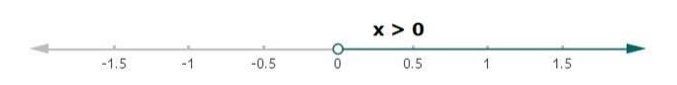# Solve each of the following in equations and represent the solution set on

Question:

Solve each of the following in equations and represent the solution set on the number line.

$\frac{2 x-1}{12}-\frac{x-1}{3}<\frac{3 x+1}{4}$ where $x \in \mathbf{R}$.

Solution:

Given:

$\frac{2 x-1}{12}-\frac{x-1}{3}<\frac{3 x+1}{4}$, where $x \in R$

Multiply by 12 on both sides in the above equation

$12\left(\frac{2 x-1}{12}\right)-12\left(\frac{x-1}{3}\right)<12\left(\frac{3 x+1}{4}\right)$

$(2 x-1)-4(x-1)<3(3 x+1)$

$2 x-1-4 x+4<9 x+3$

$3-2 x<9 x+3$

Now, subtracting 3 on both sides in the above equation

$3-2 x-3<9 x+3-3$

$-2 x<9 x$

Now, subtracting 9x from both the sides in the above equation

$-2 x-9 x<9 x-9 x$

$-11 x<0$

Multiplying -1 on both the sides in above equation

$(-11 x)(-1)<(0)(-1)$

$11 x>0$

Dividing both sides by 11 in above equation

$\frac{11 x}{11}>\frac{0}{11}$

Therefore,

$x>0$